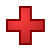Games
Problems
Go Pro!

Quizzes > Mathematics > Arithmetic > Addition

# Featured QuizzesPractice your addition facts, adding single digit numbers. Students who remember these facts do their work much more efficiently than students who...Practice your addition facts with this simple math quiz from The Problem Site.Practice your addition facts with this simple math quiz from The Problem Site.Practice your addition facts with this simple math quiz from The Problem Site.Practice your addition facts with this simple math quiz from The Problem Site.Practice your addition facts with this simple math quiz from The Problem Site.Practice your addition facts with this simple math quiz from The Problem Site.Practice your addition facts with this simple math quiz from The Problem Site.

# Full Directory ListingLike us on Facebook to get updates about new resources